# NCERT Solutions for Class 8 Maths Chapter 15 Introduction to Graphs| PDF Download

Chapter 15 Introduction to Graphs NCERT Solutions for Class 8 Maths is available on this page which is very helpful solving the difficult problems in a given in a exercise. Also, you can download PDF of Chapter 15 Introduction to Graphs NCERT Solutions which can be used to recall various questions any time. These NCERT Solutions will help an individual to increase concentration and will tell you about the difficulty of questions.

Class 8 Maths NCERT Solutions will help in developing your problem solving skills and be aware of the concepts. There are variety of concepts given in this Chapter 15 Class 9 Maths textbook that will develop your necessary skill to solve more and more questions.Page No: 236

Exercise 15.1

1. The following graph shows the temperature of a patient in a hospital, recorded every hour:
(a) What was the patient’s temperature at 1 p.m.?

(b) When was the patient’s temperature 38.5° C?
(c) The patient’s temperature was the same two times during the period given. What were these two times?
(d) What was the temperature at 1.30 p.m.? How did you arrive at your answer?
(e) During which periods did the patients’ temperature showed an upward trend?

(a) The patient’s temperature was 36.5o C at 1 p.m.
(b) The patient’s temperature was 38.5o C at 12 noon.
(c) The patient’s temperature was same at 1 p.m. and 2 p.m.
(d) The temperature at 1.30 p.m. is 36.5o C. The point between 1 p.m. and 2 p.m., x-axis is equidistant from the two points showing 1 p.m. and 2 p.m. So, it represents 01.30 p.m. Similarly, the point on y-axis, between 36o C and 37o C will represent 36.5o C.
(e) The patient’s temperature showed an upward trend from 9 a.m. to 11 a.m., 11 a. m. to 12 noon and 2 p.m. to 3 p.m.

2. The following line graph shows the yearly sales figures for a manufacturing company.
(a) What were the sales in (i) 2002 (ii) 2006?

(b) What were the sales in (i) 2003 (ii) 2005?
(c) Compute the difference between the sales in 2002 and 2006.
(d) In which year was there the greatest difference between the sales as compared
to its previous year?

(a) The sales in: (i) 2002 was Rs.4 crores and (ii) 2006 was Rs.8 crores.
(b) The sales in: (i) 2003 was Rs.7 crores (ii) 2005 was Rs.10 crores.
(c) The difference of sales in 2002 and 2006 = Rs.8 crores – Rs.4 crores = Rs.4 crores
(d) In the year 2005, there was the greatest difference between the sales as compared to its previous year, which is (Rs.10 crores – Rs.6 crores) = Rs.4 crores.

3. For an experiment in Botany, two different plants, plant A and plant B were grownunder similar laboratory conditions. Their heights were measured at the end of each week for 3 weeks. The results are shown by the following graph.
(a) How high was Plant A after (i) 2 weeks (ii) 3 weeks?
(b) How high was Plant B after (i) 2 weeks (ii) 3 weeks?
(c) How much did Plant A grow during the 3rd week?
(d) How much did Plant B grow from the end of the 2nd week to the end of the 3rd week?
(e) During which week did Plant A grow most?
(f) During which week did Plant B grow least?
(g) Were the two plants of the same height during any week shown here? Specify.

(a) (i) The plant A was 7 cm high after 2 weeks and
(ii) after 3 weeks it was 9 cm high.

(b) (i) Plant B was also 7 cm high after 2 weeks and
(ii) after 3 weeks it was 10 cm high.

(c) Plant A grew = 9 cm – 7 cm = 2 cm during 3rd week.

(d) Plant B grew during end of the 2nd week to the end of the 3rd week = 10 cm – 7 cm = 3 cm.

(e) Plant A grew the highest during second week.

(f) Plant B grew the least during first week.

(g) At the end of the second week, plant A and B were of the same height.

4. The following graph shows the temperature forecast and the actual temperature for each day of a week.
(a) On which days was the forecast temperature the same as the actual temperature?
(b) What was the maximum forecast temperature during the week?
(c) What was the minimum actual temperature during the week?
(d) On which day did the actual temperature differ the most from the forecast temperature?

(a) On Tuesday, Friday and Sunday, the forecast temperature was same as the actual temperature.
(b) The maximum forecast temperature was 35o C.
(c) The minimum actual temperature was 15o C.
(d) The actual temperature differed the most from the forecast temperature on Thursday.

5. Use the tables below to draw linear graphs.
 Year 2003 2004 2005 2006 Days 8 10 5 12
(a) The number of days a hill side city received snow in different years.
 Year 2003 2004 2005 2006 2007 No. of Men 12 12.5 13 13.2 13.5 No. of Women 11.3 11.9 13 13.6 12.8
(b) Population (in thousands) of men and women in a village in different years.

(a)(b)6. A courier-person cycles from a town to a neighbouring suburban area to deliver a parcel to a merchant. His distance from the town at different times is shown by the following graph.
(a) What is the scale taken for the time axis?

(b) How much time did the person take for the travel?
(c) How far is the place of the merchant from the town?
(d) Did the person stop on his way? Explain.
(e) During which period did he ride fastest?

(a) 4 units = 1 hour.
(b) The person took 3.1/2 hours for the travel.
(c) It was 22 km far from the town.
(d) Yes, this has been indicated by the horizontal part of the graph. He stayed from 10 am to 10.30 am.
(e) He rode the fastest between 8 am and 9 am.

7. Can there be a time-temperature graph as follows? Justify your answer.

(i) It is showing the increase in temperature.
(ii) It is showing the decrease in temperature.
(iii) The graph figure (iii) is not possible since temperature is increasing very rapidly which is not possible.
(iv) It is showing constant temperature.

Page No. 243

Exercise 15.2

1. Plot the following points on a graph sheet. Verify if they lie on a line
(a) A(4,0), B(4,2), C(4,6), D(4, 2.5)
(b) P(1,1), Q(2,2), R(3,3), S(4,4)
(c) K(2, 3), L(5, 3), M(5, 5), N(2, 5)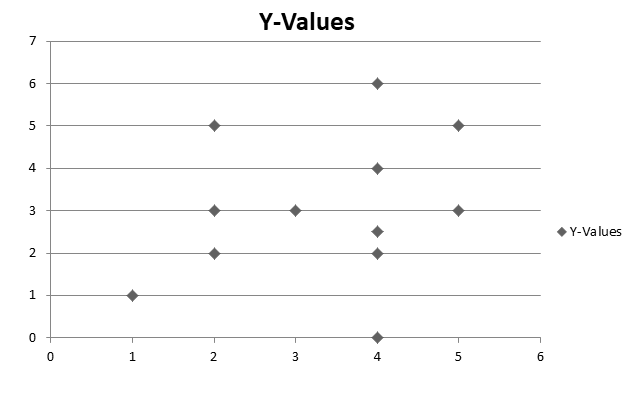(a) All points A, B, C and D lie on a vertical line.
(b) P, Q, R and S points also make a line. It verifies that these points lie on a line.
(c) These points do not lie in a straight line.

2. Draw the line passing through (2, 3) and (3, 2). Find the coordinates of the points at which this line meets the x-axis and y-axis.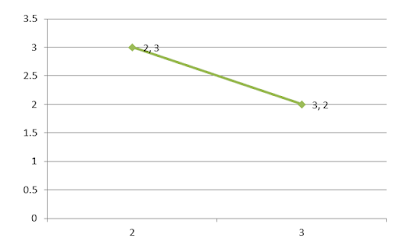The coordinates of the points at which this line meets the x-axis at (5, 0) and y-axis at (0, 5).

3. Write the coordinates of the vertices of each of these adjoining figures.Vertices of figure OABC
O (0, 0), A (2, 0), B (2, 3) and C (0, 3)
Vertices of figure PQRS
P (4, 3), Q (6, 1), R (6, 5) and S (4, 7)
Vertices of figure LMK
L (7, 7), M (10, 8) and K (10, 5)

4. State whether True or False. Correct that are false.
(i) A point whose x coordinate is zero and y-coordinate is non-zero will lie on the y-axis.
(ii) A point whose y coordinate is zero and x-coordinate is 5 will lie on y-axis.
(iii) The coordinates of the origin are (0, 0).

(i) True
(ii) False, it will lie on x-axis.
(iii) True

Page No. 247

Exercise 15.3

1. Draw the graphs for the following tables of values, with suitable scales on the axes.
(a) Cost of apples
 No. of apples 1 2 3 4 5 Cost (in Rs.) 5 10 15 20 25
(b) Distance travelled by a car
 Time (in hours) 6 a.m. 7 a.m. 8 a.m. 9 a.m. Distance (in km) 40 80 120 160
(i) How much distance did the car cover during the period 7.30 a.m. to 8 a.m?
(ii) What was the time when the car had covered a distance of 100 km since it’s start?

(c) Interest on deposits for a year.
 Deposit(inRs.) 1000 2000 3000 4000 5000 Simple Interest (inRs.) 80 160 240 320 400
(i) Does the graph pass through the origin?
(ii) Use the graph to find the interest on Rs 2500 for a year.
(iii) To get an interest of Rs 280 per year, how much money should be deposited?

(a)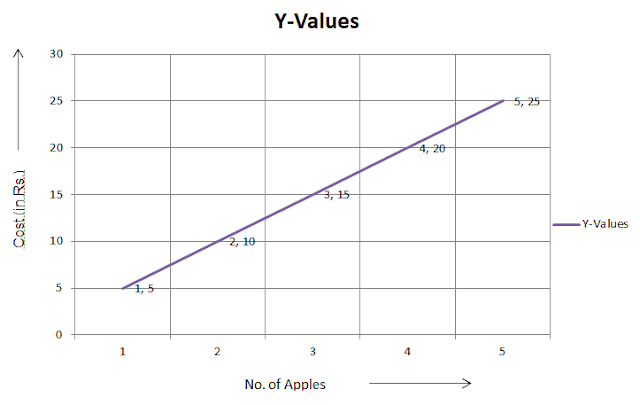(b) (i) The car covered 20 km distance.
(ii) It was 7.30 am, when it covered 100 km distance.(c) (i) Yes, the graph passes through the origin.
(ii) Interest on Rs. 2500 is Rs. 200 for a year.
(iii) Rs. 3500 should be deposited for interest of Rs. 280.2. Draw a graph for the following.
(i)
 Side of Square(in cm) 2 3 3.5 5 6 Perimeter (in cm) 8 12 14 20 24
Is it a linear graph?
(ii)
 Side of Square(in cm) 2 3 4 5 6 Area (in cm2) 4 9 16 25 36
Is it a linear graph?

(i) Yes, it is a linear graph.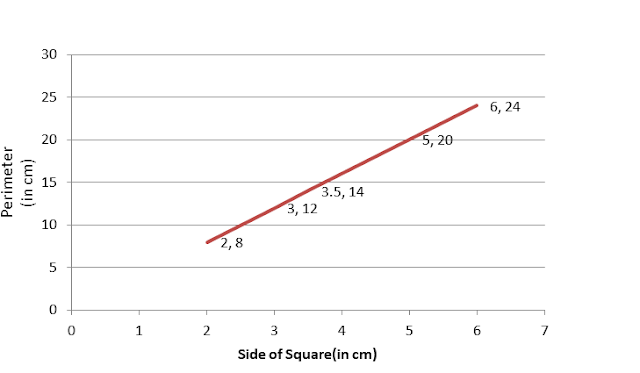(ii) No, it is not a linear graph because the graph does not provide a straight line.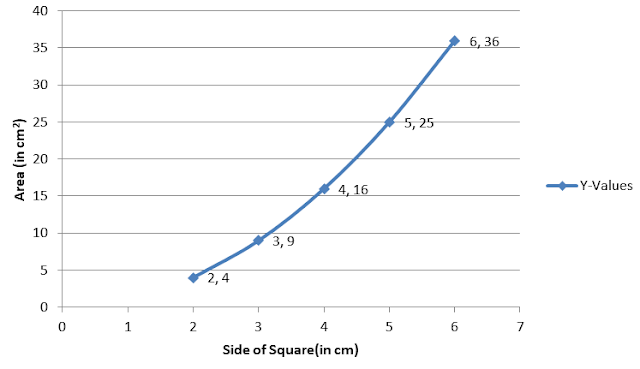## NCERT Solutions for Class 8 Maths Chapter 15 Introduction to Graphs

Chapter 15 NCERT Solutions will prove useful guide in making a student confident and developing you understanding of the chapter and make a student confident. The graphs are visual representations of data collected.

• Pictorial and graphical representation are easier of understand.

• Bar graphs and pie graphs are used for comparing categories and parts respectively.

• A histogram is a bar graph that shows data in intervals.

Exercisewise NCERT Solutions for Class 8 Maths can be really helpful if anyone want to understand the detailed solutions and minimise the errors wherever possible. Studyrankers experts have prepared accurate and detailed NCERT Solutions through which you can be able to solve more questions related to chapter.

### NCERT Solutions for Class 8 Maths Chapters:

 Chapter 1 Rational Numbers Chapter 2 Linear Equations in Variable Chapter 3 Understanding Quadrilaterals Chapter 4 Practical Geometry Chapter 5 Data Handling Chapter 6 Squares and Square Roots Chapter 7 Cubes and Cube Roots Chapter 8 Comparing Quantities Chapter 9 Algebraic Expressions and Identities Chapter 10 Visualising Solid Shapes Chapter 11 Mensuration Chapter 12 Exponents and Powers Chapter 13 Direct and Inverse Proportions Chapter 14 Factorisation Chapter 16 Playing with Numbers

FAQ on Chapter 15 Introduction to Graphs

#### Why we should solve NCERT Solutions for Chapter 15 Introduction to Graphs Class 8?

Chapter 15 Introduction to Graphs Class 8 NCERT Solutions are very essential in revising the chapter properly and build your own answers for homework and get good marks in the examination.

#### Are the coordinates (4, 5) and (5, 4) the same? Why?

No, (4, 5) and (5, 4) are not the same. In (4, 5) the x-coordinate is 4 whereas in (5, 4) it is 5. The same is with the y-coordinate too. As a result, they are different and they represent the different sets of points.

#### What do you mean by Line Graph?

A line graph displays data that change continuously over periods of time. This graph being wholly an unbroken line is called a linear graph.

#### What do you mean by Pie Graph?

A pie-graph is used to compare various parts of a whole using a circle.